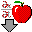# C3: Uncompetitive Inhibition

$$\newcommand{\vecs}{\overset { \rightharpoonup} {\mathbf{#1}} }$$ $$\newcommand{\vecd}{\overset{-\!-\!\rightharpoonup}{\vphantom{a}\smash {#1}}}$$$$\newcommand{\id}{\mathrm{id}}$$ $$\newcommand{\Span}{\mathrm{span}}$$ $$\newcommand{\kernel}{\mathrm{null}\,}$$ $$\newcommand{\range}{\mathrm{range}\,}$$ $$\newcommand{\RealPart}{\mathrm{Re}}$$ $$\newcommand{\ImaginaryPart}{\mathrm{Im}}$$ $$\newcommand{\Argument}{\mathrm{Arg}}$$ $$\newcommand{\norm}{\| #1 \|}$$ $$\newcommand{\inner}{\langle #1, #2 \rangle}$$ $$\newcommand{\Span}{\mathrm{span}}$$ $$\newcommand{\id}{\mathrm{id}}$$ $$\newcommand{\Span}{\mathrm{span}}$$ $$\newcommand{\kernel}{\mathrm{null}\,}$$ $$\newcommand{\range}{\mathrm{range}\,}$$ $$\newcommand{\RealPart}{\mathrm{Re}}$$ $$\newcommand{\ImaginaryPart}{\mathrm{Im}}$$ $$\newcommand{\Argument}{\mathrm{Arg}}$$ $$\newcommand{\norm}{\| #1 \|}$$ $$\newcommand{\inner}{\langle #1, #2 \rangle}$$ $$\newcommand{\Span}{\mathrm{span}}$$

Reversible uncompetitive inhibition occurs when ($$I$$) binds only to the enzyme-substrate complex ($$ES$$) and not free $$E$$. One can hypothesize that on binding S, a conformational change in $$E$$ occurs which presents a binding site for $$I$$. Inhibition occurs since $$ESI$$ can not form product. It is a dead end complex which has only one fate, to return to $$ES$$. This is illustrated in the chemical equations and molecular cartoon below.Let us assume for ease of equation derivation that $$I$$ binds reversibly to ES with a dissociation constant Kii. A look at the top mechanism shows that in the presence of $$I$$, as $$S$$ increases to infinity, not all of $$E$$ is converted to ES. That is, there is a finite amount of ESI, even at infinite $$S$$. Now remember that $$V_m = k_{cat}E_o$$ if and only if all $$E$$ is in the form ES . Under these conditions, the apparent $$V_m$$, $$V_{mapp}$$ is less than the real $$V_m$$ without inhibitor. In addition, the apparent $$K_m$$, $$K_{mapp}$$, will change. We can use Le Chatelier's principle to understand this. If $$I$$ binds to ES alone, and not $$E$$, it will shift the equilibrium of $$E + S \rightleftharpoons ES$$ to the right, which would have the affect of decreasing the $$K_{mapp}$$ (i.$$E$$., it would appear that the affinity of $$E$$ and $$S$$ has increased.).

The double reciprocal plot (Lineweaver Burk plot) offers a great way to visualize the inhibition. In the presence of $$I$$, both $$V_m$$ and $$K_m$$ decrease. Therefore, $$-1/K_m$$, the x-intercept on the plot, will get more negative, and $$1/V_m$$ will get more positive. It turns out that they change to the same extent. Therefore the plots will consist of a series of parallel lines, which is the hallmark of uncompetitive inhibition.An equation, shown in the diagram above, can be derived which shows the effect of the uncompetitive inhibitor on the velocity of the reaction. The only change is that the $$S$$ term in the denominator is multiplied by the factor $$1+I/K_{ii}$$. We would like to rearrange this equation to show how $$K_m$$ and $$V_m$$ are affected by the inhibitor, not $$S$$, which obviously is not. Rearranging the equation as shown above shows that

$K_{mapp} = \dfrac{K_m}{(1+I/K_{ii})}$ and $V_{mapp} = \dfrac{V_m}{1+I/K_{ii}}.$

This shows that the apparent $$K_m$$ and $$V_m$$ do decrease as we predicted. $$K_{ii}$$ is the inhibitor dissociation constant in which the inhibitor affects the intercept of the double reciprocal plot. Note that if $$I$$ is zero, $$K_m$$ and $$V_m$$ are unchanged.Java Applet: Uncompetitive InhibitionWolfram Mathematica CDF Player - Uncompetitive Inhibition v vs S (free plugin required)4/6/14Wolfram Mathematica CDF Player - Uncompetitive Inhibition - Lineweaver Burk(free plugin required)